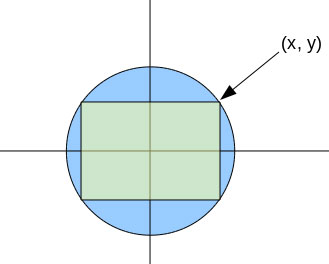SEARCH HOMEMath Central Quandaries & QueriesQuestion from Niki, a student: Consider a rectangle inscribed in a circle with a radius or R. What are the possible perimeters for the rectangle?Hi Niki.

The best way to approach this is to center the circle on the origin of a normal graph and use x as your independent variable.Now it should be clear to you that the perimeter is simply 4(x+y). Thus, we really only need to think about the first quadrant.

x varies from 0 to R, of course, and so does y. But you can express f(x) = y by re-arranging the circle's equation

R2 = x2 + y2.

Then just find the minimum and maximum values of the expression x + f(x) and multiply by four.

Write back if you need more hints.

Cheers,
Stephen La Rocque.Math Central is supported by the University of Regina and The Pacific Institute for the Mathematical Sciences.